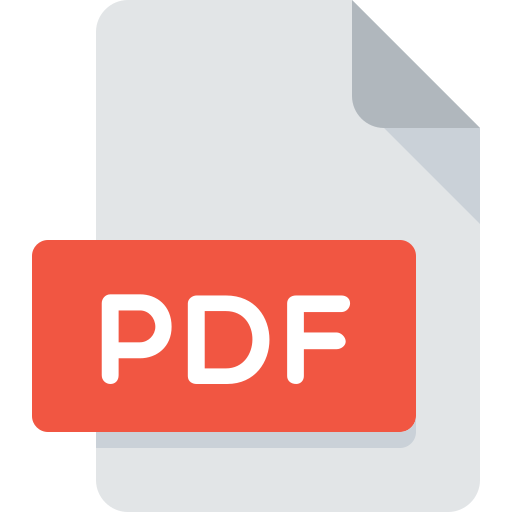Engineering (EGR) Courses

## Engineering (EGR)

### STEM (Science, Technology, Engineering, Math)

Science, Engineering, & Arch Department

### EGR 101—Introduction to Engineering Design

2 lect., 2 lab, 3 cr.

(Fall)

An introduction to Engineering as a career with emphasis on communication skills. Topics to be presented include engineering graphics, technical report writing, computer graphics, 3D graphics modeling, 2D physical modeling and introduction to spreadsheets.

### Pre/Corequisite:

MAT 121 or higher

### EGR 102—Programming for Engineers

2 lect., 2 lab, 3 cr.

(Spring)

An introduction to engineering calculations involving the use of the digital computer. A structured object-oriented language such as C++ or Java is taught. Problems are drawn from DC- AC- digital circuit theory, numerical methods. A programming language course where problems are also solved using spreadsheets, math processors circuits modeling program, and visualization applications.

### Pre/Corequisite:

MAT 121 or higher

### EGR 205—Statics

4 cr.

(Fall)

Deals with forces in static equilibrium, including frictional forces. Introduces matrices to solve equations of more than one unknown. Thorough treatment of centroids and second moments. Maximum and minimum second moments; principal axis.

### Prerequisite:

C or better in PHY 104

MAT 207

### EGR 206—Dynamics

4 cr.

(Spring)

Kinematics: absolute and relative motion. Force, mass, and acceleration. Work and energy, Impulse and momentum. Mechanical vibrations. Modern use of vector analysis throughout the course.

### Prerequisite:

C or better in EGR 205

### EGR 212—Circuit Theory

3 cr.

(Spring)

Sinusoidal analysis of circuits using complex algebra and phasor concept. Average and RMS voltage, current and power. Complex power. Fourier analysis. Series and parallel resonance. Polyphase circuits. Complex frequency. Thevenin's and Norton's Theorem. Superposition theorem.

PHY 203

### EGR 214—Thermodynamics

3 cr.

(Summer)

A study of the first and second laws of thermodynamics, open and closed energy systems, properties, and unit systems. Includes application to compressors, pumps, turbines, heat exchangers, and nozzles.

### Prerequisite:

PHY 104 (or PHY 105), MAT 206

### EGR 216—Engineering Computations

2 cr.

(Spring)

A survey of the mathematical methods used in electricity and magnetism, and mechanics. The goal of the course is to introduce the gradient divergence, curl, and Laplacian. Application to the wave equation.

MAT 207

MAT 214

### EGR 218—Materials Science

3 cr.

(Fall)

A study of the relationship between the structure and properties of metallic, organic, and ceramic compounds. The physical structure of materials and their limitations are related to use in the areas of science and engineering.

### Prerequisite:

CHM 102 (previously CHM 106), PHY 104 (or PHY 106)

### EGR 220—Solid Mechanics

3 cr.

(Spring)

Analysis of stress and strain due to axial, torsional, thermal and flextural loads; elastic deformation and buckling applied to beams, shafts and columns. The course will address statically determinant and indeterminant problems. The concepts of principal stresses, principal strains and Mohr's Circle will be presented as well as well as shear and moment diagrams.

### Prerequisite:

EGR 205, MAT 207Download PDF